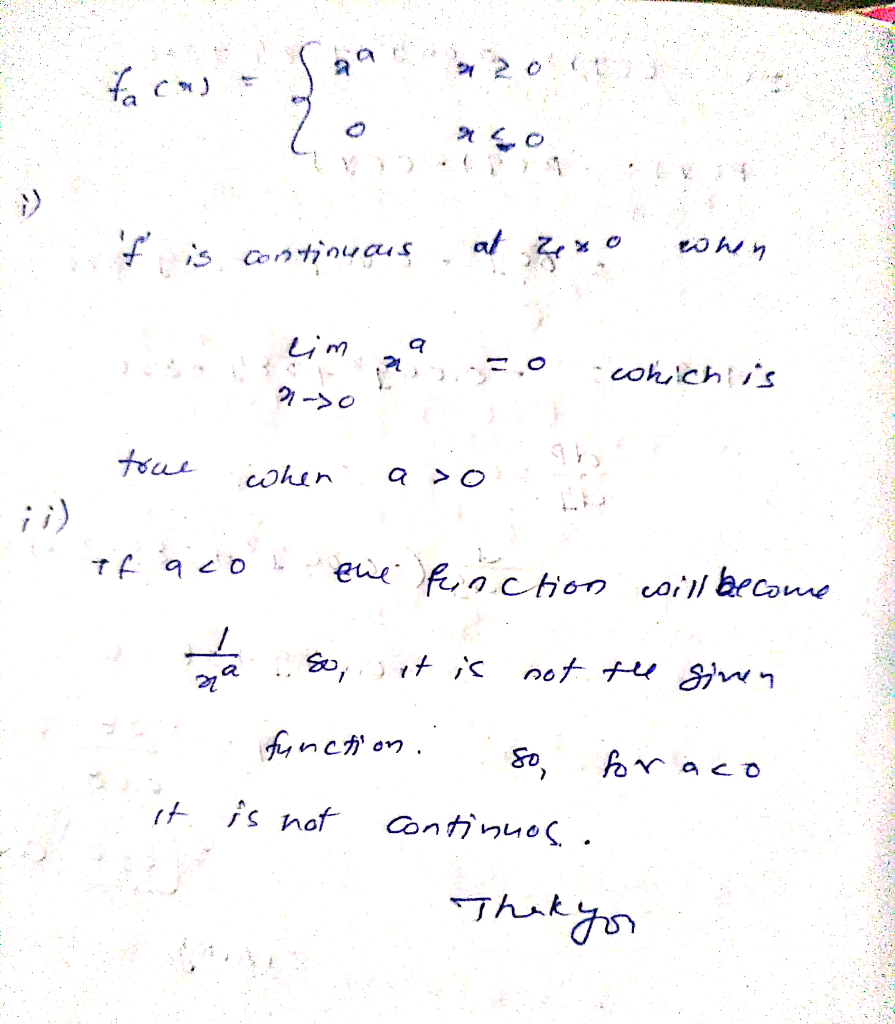Homework Help Question & Answers

Let fa(x)= x^a if x>0, 0 if x<0. a) for what values of a is f continuous at 0? WHY is a<...

Let fa(x)= x^a if x>0, 0 if x<0.

a) for what values of a is f continuous at 0?

WHY is a<0 not continuous at 0? Please explain.Add Answer of: Let fa(x)= x^a if x>0, 0 if x<0. a) for what values of a is f continuous at 0? WHY is a<...
More Homework Help Questions Additional questions in this topic.

• Let D be a Euclidean domain values? (That is, must the range of v be infinite?) Let D be a Euclidean domain values? (That is, must the range of v be infinite?)

Need Online Homework Help?

Get FREE EXPERT Answers
WITHIN MINUTES
Related Questions